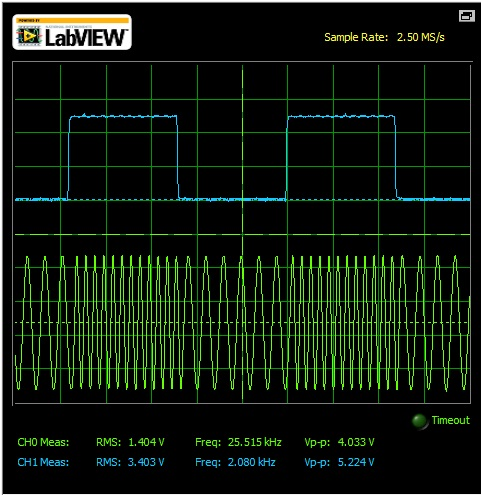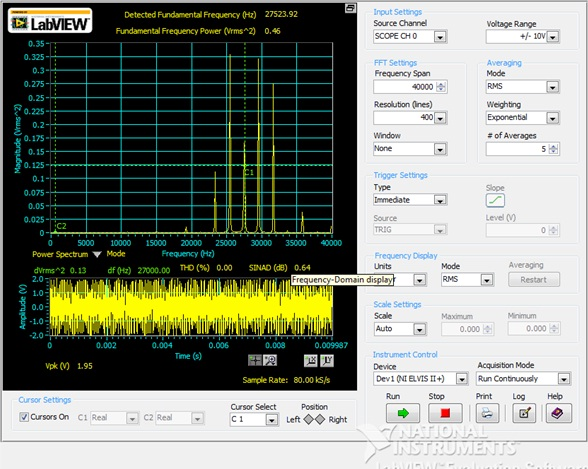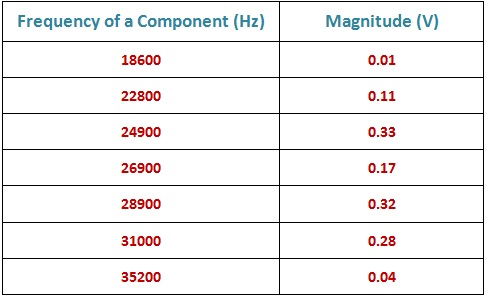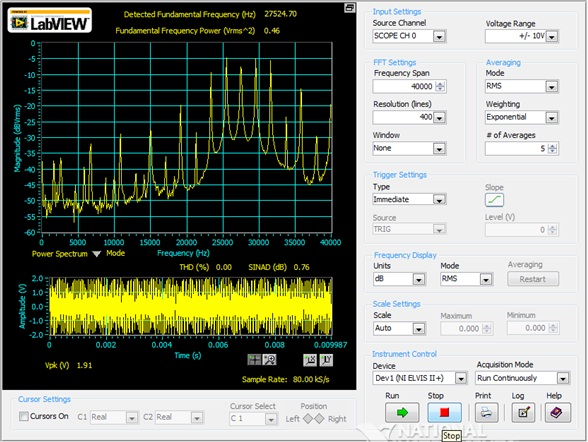Frequency Shift Keying Signal

Calculations & ObservationsFig.1 Showing FSK Modulation carried out of digital signal.

In the figure above, there is FSK( Frequency Shift Keying) Modulation of digital signal, there are two frequencies will exits as explained earlier. The two frequencies are :

1. The frequency of the signal that corresponds with logic - Os in the digital data called the space frequency is usually lower than the modulator's nominal carrier frequency.
2. The space frequency is 25252.52 Hz.
3. The frequency of the signal that corresponds with logic-ls in the digital data called the mark frequency is usually higher than the modulator's nominal carrier frequency.
4. The mark frequency is 48076.92 Hz.

The scope CH1 showing the O / p of Sequence Generator.Fig.2 Frequency Spectrum of FSK Modulation.

In the above figure 2, the Fundamental frequency detected is at 27523.92 Hz & the Fundamental Frequency Power is 0.46. The THD is 0% & SINAD is 0.64 dB.

The Dominant Frequency components presents with their magnitude are tabulated below :Fig.3 Power Spectrum in dB scale.

In the figure 3, the Power Spectrum is showed in the dB scale in which there is THD is 0% & the SINAD is 0.76 dB. The fundamental frequency present is at 27534.70 Hz & the Fundamental Frequency Power is 0.46.